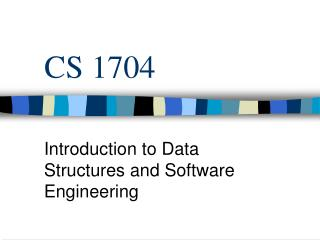DownloadDownload PresentationCS 1704

# CS 1704

Télécharger la présentation## CS 1704

- - - - - - - - - - - - - - - - - - - - - - - - - - - E N D - - - - - - - - - - - - - - - - - - - - - - - - - - -
##### Presentation Transcript

1. CS 1704 Introduction to Data Structures and Software Engineering

2. Const Pointers as Function Parameters • Four ways to use const with pointers and functions • Non-constant pointer to non-constant data • Data can be manipulated and pointer can change • Non-constant pointer to constant data • Pointer can be made to point to something else, data cannot change • Constant pointer to non-constant data • Data can be changed, pointer cannot • Constant pointer to constant data • Nothing can change •  Simple, Right?

3. Examples //pointer to a const char void printCharacters (const char *sPtr ) { for ( ; *sPtr != ‘\0’; sPtr++ ) cout << *sPtr; }

4. More Examples int main() { int x, y; int * const ptr = x; //const pointer to an int *ptr = 7; ptr = &y; return 0; }

5. One more example int main() { int x=5,y; const int * const ptr = &x; //const pointer to a // const int *ptr = 7; ptr = &y; return 0; }

6. Pointer Expressions and Pointer Arithmetic • You can: • Increment • Decrement • Add • Subtract • Compare

7. Void * • void * is a pointer to any type of data • It should be avoided unless necessary • A pointer of any type can be cast to a void * • You cannot dereference a void * • You must first cast the void * to the type of pointer it is, then dereference

8. Array Pointer • Assume we have int b and int * bPtr • We can do this: • bPtr = b; • bPtr = &b[ 0 ]; • Also, for example b is: • ( bPtr + 3 ) • *( b + 3 )

9. Arrays of Pointers • Consider the following declartion: • const char *suit = { “Hearts”, “Diamonds” “Clubs”, “Spades” }; • How does this compare with a two dimensional array that would normally have to hold these strings?

10. Dynamic Data  • The programmer can if they wish create an object dynamically. • Meaning rather than using memory given to the program when it begins and resides in its memory space • You can get it from the system heap.

11. Syntax • You use the command new • Time *timePtr; • timePtr = new Time; • timePtr2= new Time(1,2,1980); • new returns a pointer to the memory allocated for the newly created object of type Time. • #include <new> to use the new standard

12. More examples • You can do this for any built-in or user-defined type • int *xPtr = new int; • You can also create an array this way • int xArrayPtr = new int [ 10 ]; • This creates an array of size ten and you access it through xArrayPtr

13. Freeing memory • To release the memory pointed to by your pointer you use the command delete • delete xPtr; • delete [ ] xPtr; • Forgetting the [ ] on an array only releases the memory for the first location in the array